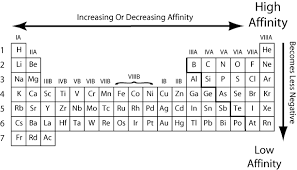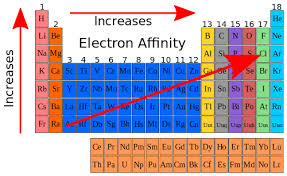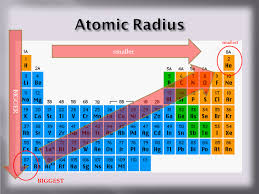# Introduction of Electron Affinity Trend, Definition & Equation

If you are a science student then it is very important for you to know about Electronic Affinity Trend and it’s equation. According to chemistry and quantum physics, electron affinity is known as the change in energy level of a neutral atom when another electron is added to it in its gaseous state. The resultant atom formed is a negative ion.

The electron affinity increases across the periodic table. Its equation is as follows :

X + e− → X− + energy

Why Does Electron Affinity Increase Across a Period?Electron affinity increases upward for the groups and from left to right across periods of a periodic table since the electrons added to the increasing proximity between the nucleus and the electrons, thus increasing the bond between the nucleus and electron. If an electron is added outside the orbital, then its affinity also decreases, since greater the distance, lesser the attraction.

Also, the number of valence electrons affects the electron affinity of an element. An element with more valence electrons would more likely to have more electron affinity than an element with lesser valence electrons.

It is so because as one goes from right to left across the periodic table, the size of the atom increase, thus increasing the radius between the nucleus and the electron. This leads to the decrease in the ability of the nucleus to pull the electrons. Thus the level of energy change decreases and this leads to lower electron affinity.

Electron Affinity Trend ExplainedThe electron affinity is a measure of an amount of attraction between an additional electron and the nucleus. It is the quantitative measurement of the energy expended when an electron is added to the atom. The first electron affinity is the amount of energy released when 1 mole of gaseous atoms acquire an electron to form 1 mole of gaseous ions in the negative state.

Since electronic affinity is a chemical reaction when an electron is introduced in an atom, there can be two types of reactions possible. The first is the endothermic reaction and the second is the exothermic reaction.

When an electron is added to a neutral atom during the first affinity process, heat energy is released. Hence this is an exothermic reaction. But during the second affinity process, more energy is required to introduce an electron to a negative ion. Hence the resultant chemical reaction is endothermic one.

Electron Affinity Trend in Periodic TableAs we go from left to right across a periodic table, the electronic affinity increases. This is because the size of the atoms decreases, hence the electrons which are added are closer to the nucleus. This results in a stronger chemical reaction and the increase in electronic affinity.

As one goes from down to up across a periodic table, the electronic affinity decreases. This is because of the increase in the size of the atoms, which results in an increasing distance between the incoming electrons and the nucleus. Hence the chemical reaction between the electrons and the nucleus is weaker, which results in a decrease in electronic affinity of the element.